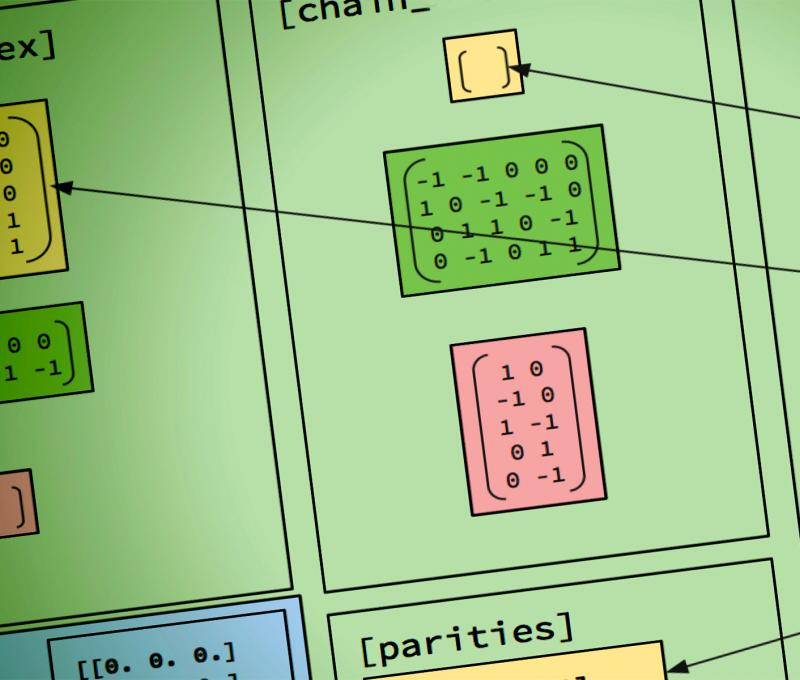# Computational Mathematical Modeling (Advanced Certificate)Offered On-Campus Only

The Advanced Certificate in Computational Mathematical Modeling (CMM) provides education and training for students with a BS in mathematics, physics, engineering, or a comparable program for careers in science and industry as well as for further graduate study in the area of physically based computational mathematical modeling and data analysis. It stresses fundamentals and applications equally. Traditionally science emerged as the interplay between theory and experiment, but increasingly computational modeling helps by simulating experiments, which cannot be done or are too expensive or not safe. Simultaneously advances in sensor technology generate large amounts of data, which can be utilized to help improve mathematical models. This approach calls for advanced computational and statistical methods.

### Students will learn how to:

• Model, analyze and solve problems from science and engineering using advanced methods from linear algebra, differential equations, and computational mathematics.

• Implement numerical methods for the solution of standard partial and ordinary differential equations, to analyze their mathematical behavior (consistency and stability), and to choose the appropriate method for different types of problems.

• Apply their knowledge of computational mathematical modeling in their area of interest, for example, fluids, elasticity, electromagnetism, quantum mechanics, material science, probability, stochastic modeling, data analysis, nanoscience or biomedical applications.

Andrea Dziubek, Ph.D.
Assistant Professor & Computational Mathematical Modeling Program Coordinator
Phone: (315) 792-7329
Email: dziubea@sunypoly.eduClick 'Fullscreen' or zoom in to view.